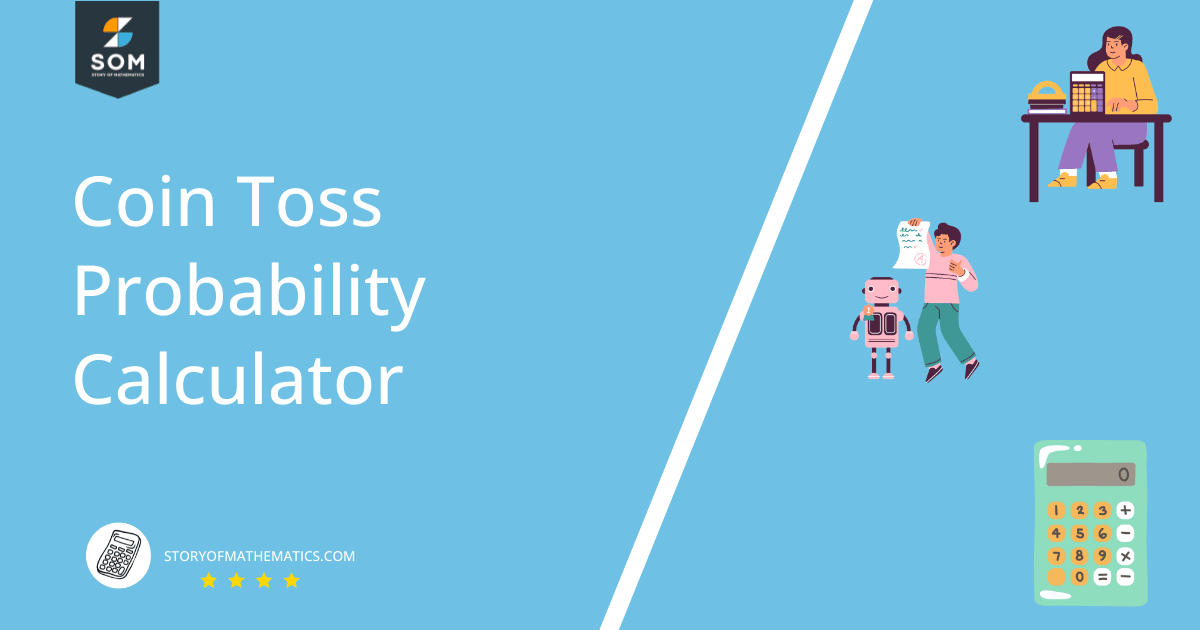# Coin Toss Probability Calculator + Online Solver With Free Steps

The Coin Toss Probability Calculator calculates the probability that exactly k heads appear in n coin tosses, where k and n are inputs.## What Is the Coin Toss Probability Calculator?

The Coin Toss Probability Calculator is an online tool that finds the probability of a certain number of heads coming up in a specified number of coin tosses, assuming a fair coin. It uses the concept of Binomial distribution to perform its calculations.

The calculator interface consists of two text boxes in a single, descriptive line of text. It reads “Probability of getting <input 1> heads in <input 2> coin tosses,” where you must enter values into inputs 1 and 2.

Assuming the desired outcome is heads, the first input is the number of coin tosses that came up heads, and the second is the total number of coin tosses.

In probability terms, the first input is the number of desired outcomes, while the second is the number of experiments or trials.

## How To Use the Coin Toss Probability Calculator?

You can use the Coin Toss Probability Calculator to find the probability that a specific, finite number of heads appear in several coin tosses.

For example, suppose you want to find the probability that out of 100 coin tosses, only one comes up as heads. With the help of the following step-by-step guideline, you should be able to use the calculator properly to solve this and other problems.

### Step 1

Enter the desired number of coin tosses that come up heads into the first text box (on the left). For our mock example, you would enter “1” here without quotes.

### Step 2

Enter the total number of coin tosses into the second text box (on the right). You would enter “100” without quotes here for the example.

### Step 3

Press the Submit button to get the results.

### Results

The results show up in a new window and contain a total of three sections.

The first section is labeled “Input Interpretation” and consists of a table detailing the experiments: no. of coin tosses, heads, and tails. You can use it to verify that the calculator is working as intended.

The second section is labeled “Probability of Occurrence” and contains the probability of the desired result. A “Show Computation” prompt is on the top right corner of this section. Clicking it will show the calculation steps (binomial distribution formula).

The third section is labeled “Stated Frequencies,” a table representing the fractions: heads/tosses and tails/tosses. For example, if we have four coin heads in 10 coin tosses, the fractions in this table are 4/10, and (10-4)/10 = 6/10 (notice their sum is 1).

For our mock example, the results are below.

• #### Input Interpretation

 coins number of flips 100 number of heads 1 number of tails 99
• #### Probability of Occurrence

$7.88861 \times 10^{-29} \approx \frac{1}{1.268 \times 10^{28}}$

With “Show Computation” selected:

$\begin{pmatrix} 1+99 \\ 1 \end{pmatrix} 2^{-(1+99)} = \frac{(1+99)!}{1! \times 99! \times 2^{1+99}} \approx 7.889 \times 10 ^{-29} \approx \frac{1}{1.268 \times 10^{28}}$

• #### Stated Frequencies

 Fraction of heads $\displaystyle\mathsf{\frac{1}{100} = 0.01}$ Fraction of tails $\displaystyle\mathsf{\frac{99}{100} = 0.99}$

## How Does the Coin Toss Probability Calculator Work?

The Coin Toss Probability Calculator works by applying the concepts of binomial probability distributions to the scenario of fair coin tosses. If n is the number of total coin tosses and k is the number of heads, the calculator uses the following formula:

$P(h=k) = \begin{pmatrix} n \\ k \end{pmatrix} 2^{-((n-k)+k)} = \begin{pmatrix} n \\ k \end{pmatrix} 2^{-n} \tag*{(1)}$

$\begin{pmatrix} n \\ k \end{pmatrix} = {}^{n}C_{k} = \frac{n!}{k!(n-k)!}$

Here, ${}^{n}C_{k}$ is the combination of n things taken k at a time. It represents the possible combinations of tosses in which heads come up k times.

A fair coin toss is a Bernoulli trial. That is, there are only two possible outcomes: heads or tails. With a fair coin, the probability of both outcomes is the same at 0.5 (50% chance for either). A sequence of independent, repeated Bernoulli trials results in a binomial distribution.

We can derive the formula in equation (1) from the general form of the binomial distribution (the random variable X is the number of heads):

$P(X = k) = \begin{pmatrix} n \\ k \end{pmatrix} \, p^k \, \left(1-p\right)^{n-k}$

Where p is the probability of the desired outcome and 1 – p is the probability of the undesired outcome (sometimes denoted q). In case of fair coins, p = 1/2 = q = 1 – 0.5 = 1/2. In that case, the expression simplifies to:

$P(X = k) = \begin{pmatrix} n \\ k \end{pmatrix} \, p^k \, q^{n-k}$

$P(X = k) = \begin{pmatrix} n \\ k \end{pmatrix} \left( \frac{1}{2} \right)^{\!\!k} \left( \frac{1}{2} \right)^{\!\!n-k}$

Or we can take the reciprocal of the fractions and reverse the power’s sign:

$P(X = k) = \begin{pmatrix} n \\ k \end{pmatrix} \left(2\right)^{-k} \, \left(2\right)^{-n+k}$

Since multiplying the same base adds the powers:

$P(X = k) = \begin{pmatrix} n \\ k \end{pmatrix} 2^{-n}$

That is the form used by the calculator! Remember it is only valid for fair coins.

## Solved Examples

### Example 1

In an experiment where we toss a fair coin ten times, what is the probability that we get heads exactly three times? What is the probability we get tails exactly five times?

### Solution

Since we have a fair coin, p = 1 – p = 0.5. Putting n = 10 and k = 3, we evaluate equation (1):

$P(h=3) = \begin{pmatrix} 10 \\ 3 \end{pmatrix} 2^{-10}$

$P(h=3) = \frac{10!}{3! \times 7!} \times \frac{1}{1024}$

$P(h=3) = \frac{120}{1024} = \frac{15}{128} = \boldsymbol{0.1171875}$

Since the coin is fair, we can use the same formula for checking the probability of tails:

$P(t=5) = \begin{pmatrix} 10 \\ 5 \end{pmatrix} 0.5^{-10}$

$P(t=5) = \frac{10!}{5! \times 5!} \times \frac{1}{1024}$

$P(t=5) = \frac{252}{1024} = \frac{63}{256} = \boldsymbol{0.24609375}$